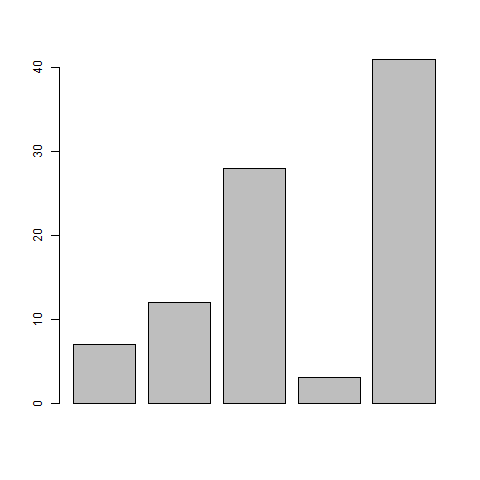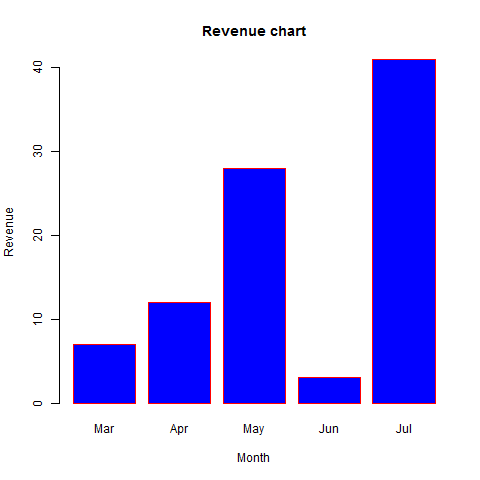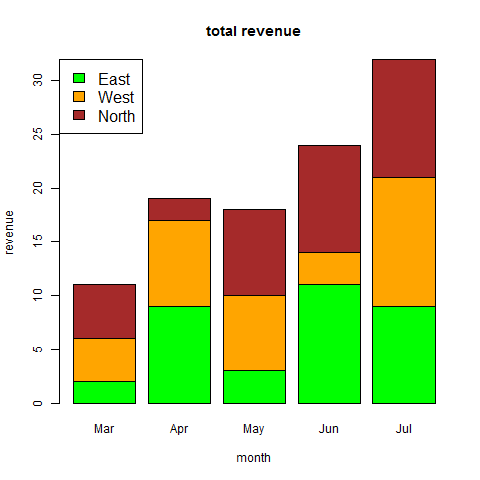### R在线运行

`极速运行模式，更高效的运行，点击编辑器上方的运行按钮即刻体验吧。`

# 条形图.

## 条形图

### 语法

``````barplot(H, xlab, ylab, main, names.arg, col)
``````

• H是包含在条形图中使用的数值的向量或矩阵。

• xlab是x轴的标签。

• ylab是y轴的标签。

• main是条形图的标题。

• names.arg是在每个条下出现的名称的向量。

• col用于向图中的条形提供颜色。

#### 例:

``````# Create the data for the chart.
H <- c(7,12,28,3,41)

# Give the chart file a name.
png(file = "barchart.png")

# Plot the bar chart.
barplot(H)

# Save the file.
dev.off()
``````### 条形图标签，标题和颜色

#### 例

``````# Create the data for the chart.
H <- c(7,12,28,3,41)
M <- c("Mar","Apr","May","Jun","Jul")

# Give the chart file a name.
png(file = "barchart_months_revenue.png")

# Plot the bar chart.
barplot(H,names.arg = M,xlab = "Month",ylab = "Revenue",col = "blue",
main = "Revenue chart",border = "red")

# Save the file.
dev.off()
``````## 组合条形图和堆积条形图

``````# Create the input vectors.
colors <- c("green","orange","brown")
months <- c("Mar","Apr","May","Jun","Jul")
regions <- c("East","West","North")

# Create the matrix of the values.
Values <- matrix(c(2,9,3,11,9,4,8,7,3,12,5,2,8,10,11),
nrow = 3,ncol = 5,byrow = TRUE)

# Give the chart file a name.
png(file = "barchart_stacked.png")

# Create the bar chart.
barplot(Values,main = "total revenue",names.arg = months,
xlab = "month",ylab = "revenue",col = colors)

# Add the legend to the chart.
legend("topleft", regions, cex = 1.3, fill = colors)

# Save the file.
dev.off()
``````JSRUN提供的R在线运行，R 在线运行工具，基于linux操作系统环境提供线上编译和线上运行，具有运行快速，运行结果与常用开发、生产环境保持一致的特点。

• 00:23